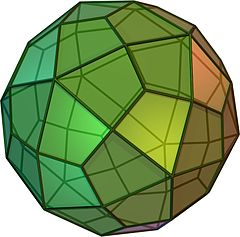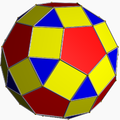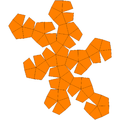Deltoidal hexecontahedron

﻿
Deltoidal hexecontahedron
Deltoidal hexecontahedronClick on picture for large version
spinning version
Type Catalan
Face polygon kite
Faces 60
Edges 120
Vertices 62 = 12 + 20 + 30
Face configuration V3.4.5.4
Symmetry group Ih, [5,3]+, 532
Dihedral angle 154° 8'
Properties convex, face-transitiverhombicosidodecahedron
(dual polyhedron)Net

In geometry, a deltoidal hexecontahedron (also sometimes called a trapezoidal hexecontahedron, a strombic hexecontahedron, or a tetragonal hexacontahedron) is a catalan solid which looks a bit like an overinflated dodecahedron. It is sometimes also called the trapezoidal hexecontahedron or strombic hexecontahedron. Its dual polyhedron is the rhombicosidodecahedron.

The 60 faces are deltoids or kites (not trapezoidal). The short and long edges of each kite are in the ratio 1.00:1.54.

It is the only Archimedean dual which does not have a Hamiltonian path among its vertices.